# Spline

A function $s_m(\Delta_n;x)$ which is defined and has continuous $(m-1)$-st derivative on an interval, and which coincides on each intervalformed by the partition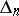: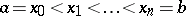with a certain algebraic polynomial of degree at most. Splines can be represented in the following way: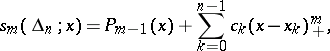where the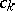are real numbers,is a polynomial of degree at most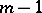, and. The points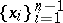are called the knots of the spline. If a splinehas a continuous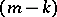-th derivative onforand at the knots the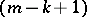-st derivative of the spline is discontinuous, then it is said to have defect. Besides these polynomial splines, one also considers more general splines (-splines), which are "tied together" from solutions of a homogeneous linear differential equation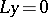, splines (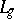-splines) with different smoothness properties at various knots, and also splines in several variables. Splines and their generalizations often occur as extremal functions when solving extremum problems, e.g. in obtaining best quadrature formulas and best numerical differentiation formulas. Splines are applied to approximate functions (see Spline approximation; Spline interpolation), and in constructing approximate solutions of ordinary and partial differential equations. They can also be used to construct orthonormal systems with good convergence properties.